Courses

# Short Answer Questions - Consumer's Equilibrium and Demand (Theory of Consumer Behaviour) Commerce Notes | EduRev

## Commerce : Short Answer Questions - Consumer's Equilibrium and Demand (Theory of Consumer Behaviour) Commerce Notes | EduRev

The document Short Answer Questions - Consumer's Equilibrium and Demand (Theory of Consumer Behaviour) Commerce Notes | EduRev is a part of the Commerce Course Economics Class 12.
All you need of Commerce at this link: Commerce

Q. Explain the five degrees of elasticity of demand?

Ans.

1. Perfectly inelastic demand: - Even with change in price, there is no change in the quantity demanded, the demand is said to be perfectly inelastic Ed =0. The demand curve is parallel to OY axis.
2. Perfectly elastic demand: - Even with no change in price there is a great change in qty.  Demanded, then the demand is said to be perfectly elastic.  The demand curve is parallel to Ox axis.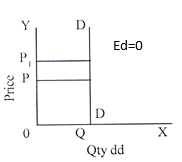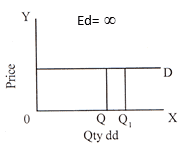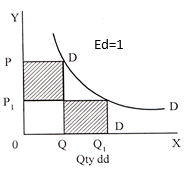3. Unitary elastic demand: When proportionate change in quantity demanded is equal to proportionate change in price, Ed is equal to unity.The demand curve resembles a rectangular hyperbola.
4. Relatively less elastic: When proportionate change in quantity demanded is less than  proportionate change in price, Ed is less than unity.
5. Relatively more elastic: When proportionate change in quantity demanded is more than  proportionate change in price, Ed is greater than unity.
6. When proportionate change in quantity demanded is less than  proportionate change in price, Ed is less than unity.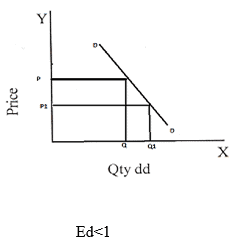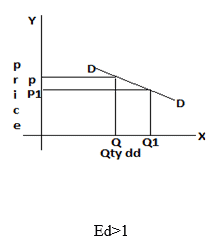Methods of Measuring Price Elasticity of Demand:-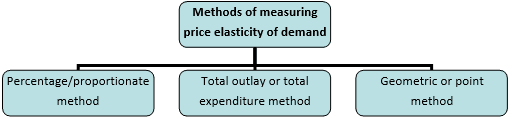Proportionate / Percentage Method: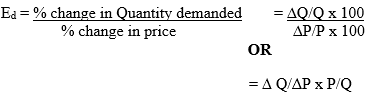Q. The Price of ice cream is Rs.20 per cup and demand is for 200 cup. If the price of ice cream falls to Rs.15 demand increases to 300 cups. Calculate elasticity of demand.

Sol.: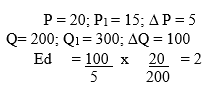Total Outlay Method (Expenditure Method)

If with the fall in price, total outlay increases elasticity of demand is greater than one, if total outlay remain constant, elasticity is equal to one and if the total outlay decreases elasticity is less than one.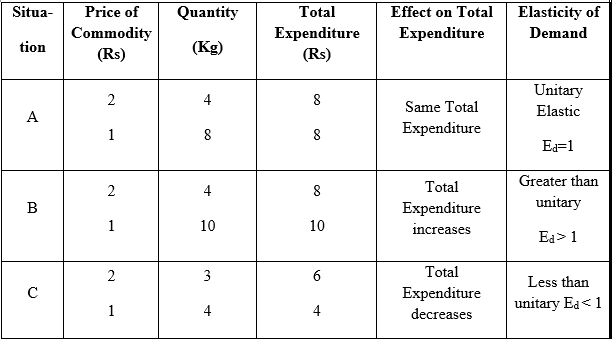Geometric / Point Method: -

This measures the elasticity of demand at different points on the same demand Curve.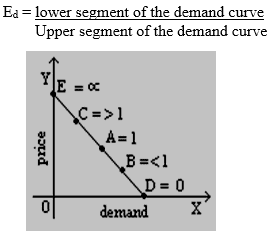ONE MARK QUESTIONS AND ANSWERS

1. What do you mean by utility?

Ans :- Utility is the want satisfying power of a commodity.

2. How is total utility derived from marginal utility?

Ans :-  Total utility is the sum total of marginal utilities of various units of a commodity.

TUn= MU1+MU2+MU3------ +MUn

3. State the law of equi-marginal utility.

Ans :- It states that a consumer gets maximum satisfaction when the ratio of the marginal utilities of two goods and their prices is equal i.e., MUx / Px = MUy / Py

4. What will you say about MU when TU is maximum?

Ans :- MU is zero when TU is maximum

5. Give the reason behind a convex indifference curve.

Ans :-  Diminishing marginal rate of substitution.

3 AND 4 MARKS QUESTIONS & ANSWERS

1. Explain the law of Diminishing Marginal Utility with the help of a table and a diagram.

Ans: - The law of diminishing Marginal Utility states that as we consume more and more units of a commodity, the MU derived from the successive units of that commodity goes on decreasing. It is explained with the help of following schedule and diagram.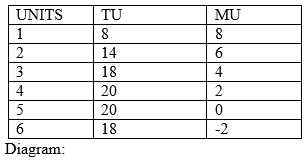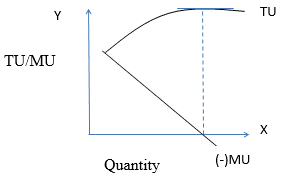Relationship between MU and TU :

i) When MU is positive TU rises.

ii) When MU is zero TU is maximum.

iii) When MU is negative, TU falls.

1. What is meant by consumer’s equilibrium? State its conditions in case of two commodities approach.

a. Meaning: A consumer is to be equilibrium when he is spending his given income on various goods and services to get maximum satisfaction.

b. Conditions:
i. MUx / Px = MUy / Py (MUs are equal to their prices)
ii. PxQx+ PyQy =M
iii. M ( Money spent is equal to income)

2. What is the difference between cardinal and ordinal utility analysis.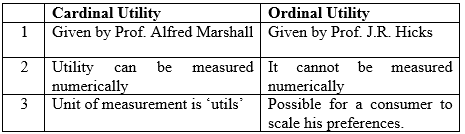3. Explain any four determinants of demand for a commodity.

Ans :-

Following are the three determinants of demand for a commodity.
Price of the commodity:- When the price of a commodity increases the demand for that commodity decreases and vice versa.
Income of the consumer:- When the income increases the demand for normal  commodity also increases and vice-versa.
Price of related goods :-
In complementary goods demand rises with fall in price of complementary goods.
In substitute goods demand for a commodity falls with a fall in the price of other substitute goods
Taste & preference of the consumer: With favourable taste, demand increase and unfavourable taste demand decreases for a commodity.

4. Draw
a) perfectly elastic demand curve,
b) perfectly inelastic demand curve and
c) unitary elastic demand curve.

Ans :- a)
perfectly elastic demand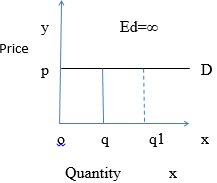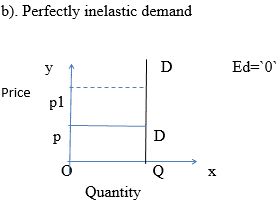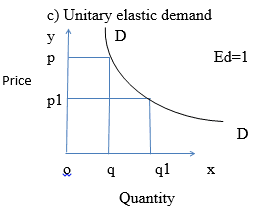6.  Explain any four factors that affect elasticity of demand.

Ans :- Following are the factors affecting price elasticity of demand.
Availability of close substitutes: If close substitutes of product are available, the commodity tends to be more elastic, If there are not available, they tend to be less elastic.

Proportion of total expenditure spent on the product-If the amount spent on a product constitutes a very small fraction of the total expenditure, then the demand tends  to be less elastic of the amount spent is high the elasticity of demand  tends to be high.

Habits: A commodity if it forms an essential part of the individual, the demand tends to be inelastic.  It is consumed casually; the demand tends to be elastic

Time period :- Longer the time period, the more elastic is the demand for any product the shorter the time period, less elastic is the demand for any products

Offer running on EduRev: Apply code STAYHOME200 to get INR 200 off on our premium plan EduRev Infinity!

## Economics Class 12

223 videos|241 docs|54 tests

,

,

,

,

,

,

,

,

,

,

,

,

,

,

,

,

,

,

,

,

,

;